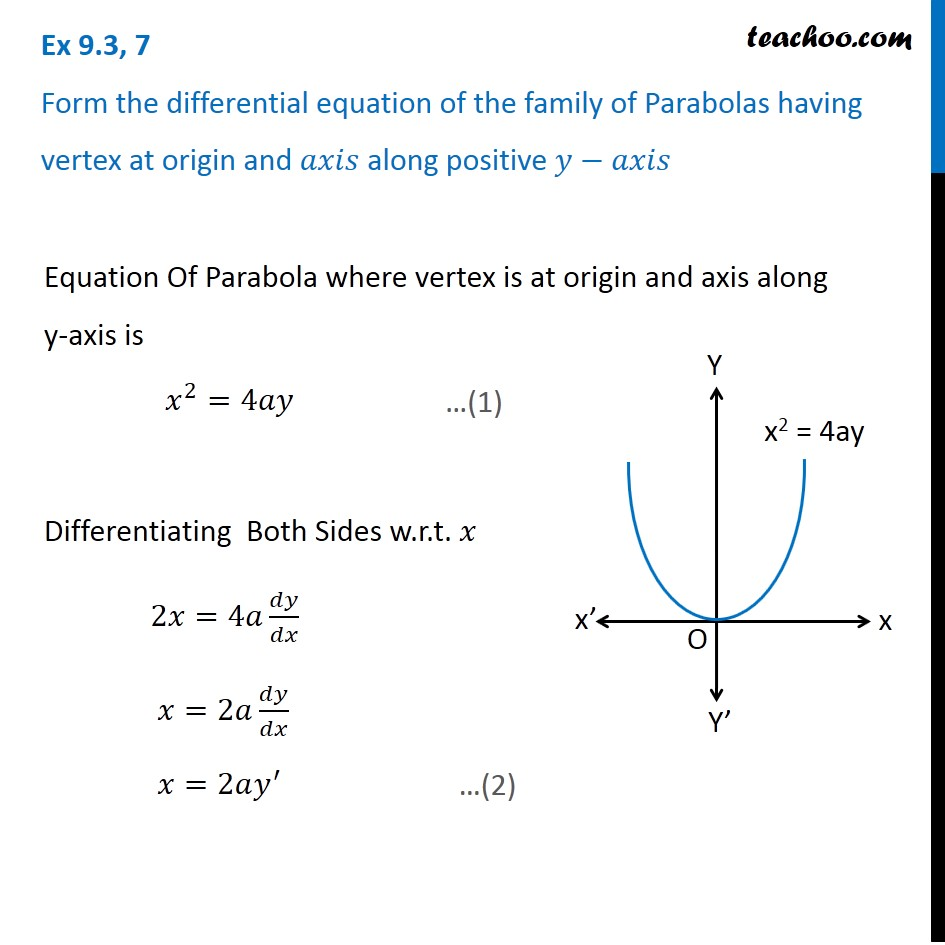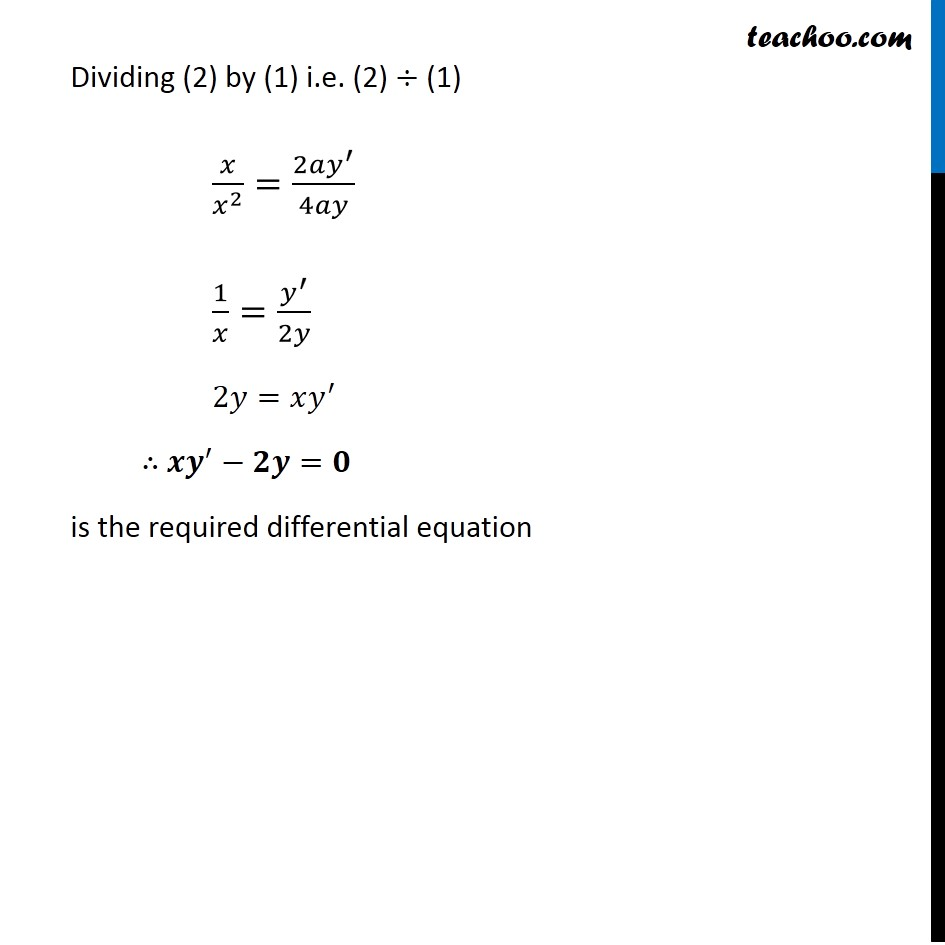Forming Differential equations

Chapter 9 Class 12 Differential Equations
Serial order wiseLearn in your speed, with individual attention - Teachoo Maths 1-on-1 Class

### Transcript

Question 7 Form the differential equation of the family of Parabolas having vertex at origin and 𝑎𝑥𝑖𝑠 along positive 𝑦−𝑎𝑥𝑖𝑠 Equation Of Parabola where vertex is at origin and axis along y-axis is 𝑥^2=4𝑎𝑦 Differentiating Both Sides w.r.t. 𝑥 2𝑥=4𝑎 𝑑𝑦/𝑑𝑥 𝑥=2𝑎 𝑑𝑦/𝑑𝑥 𝑥=2𝑎𝑦^′ Dividing (2) by (1) i.e. (2) ÷ (1) 𝑥/𝑥^2 =(2𝑎𝑦^′)/4𝑎𝑦 1/𝑥=𝑦^′/2𝑦 2𝑦=𝑥𝑦^′ ∴ 𝒙𝒚^′−𝟐𝒚=𝟎 is the required differential equation